Скачать презентацию Introduction to Abstract Interpretation Neil Kettle Andy King

f5cfcbbea141bc031954c678f33bc218.ppt

• Количество слайдов: 66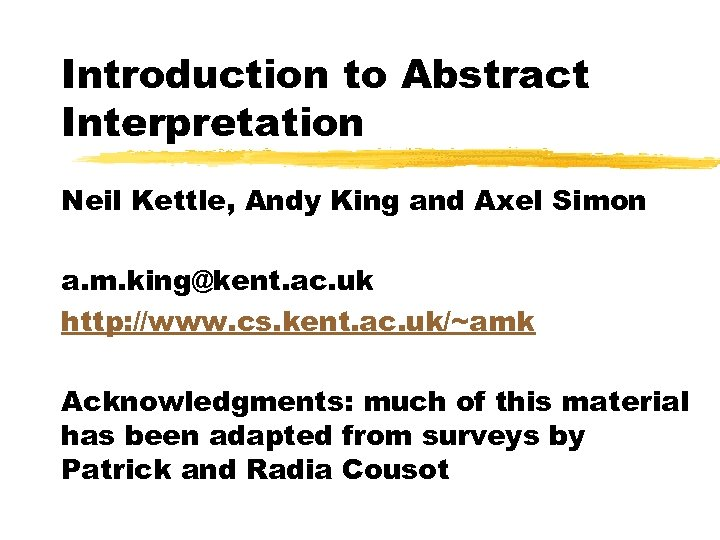Introduction to Abstract Interpretation Neil Kettle, Andy King and Axel Simon a. m. [email protected] ac. uk http: //www. cs. kent. ac. uk/~amk Acknowledgments: much of this material has been adapted from surveys by Patrick and Radia Cousot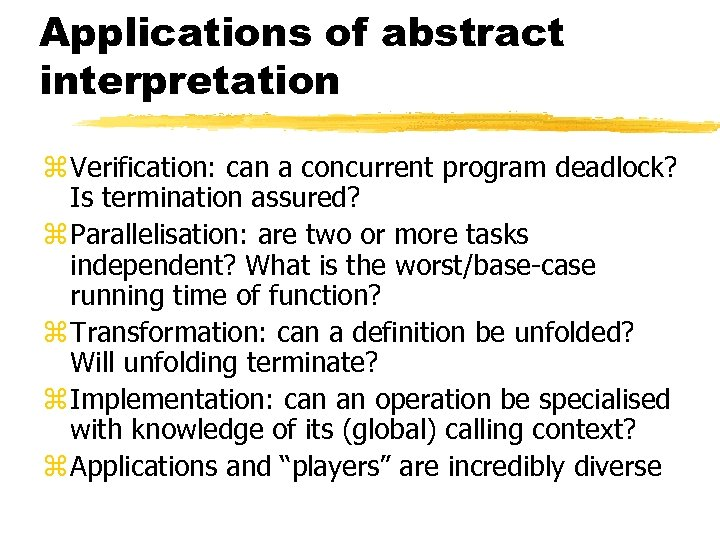Applications of abstract interpretation z Verification: can a concurrent program deadlock? Is termination assured? z Parallelisation: are two or more tasks independent? What is the worst/base-case running time of function? z Transformation: can a definition be unfolded? Will unfolding terminate? z Implementation: can an operation be specialised with knowledge of its (global) calling context? z Applications and “players” are incredibly diverse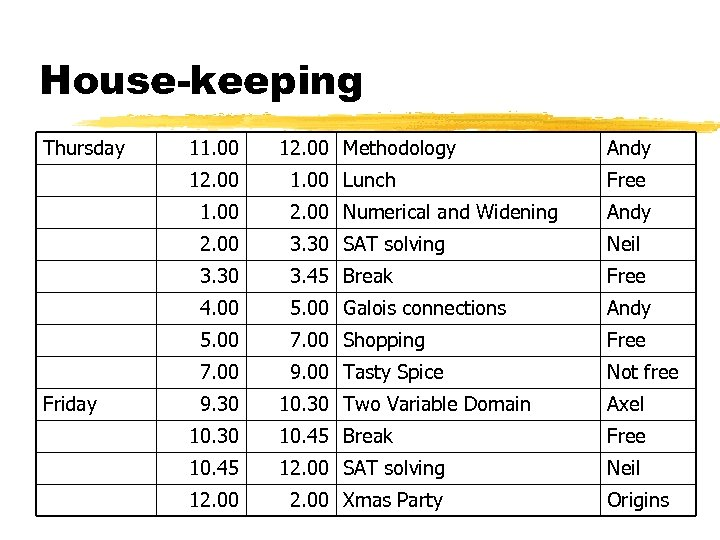House-keeping Thursday 11. 00 12. 00 Methodology Andy Free 1. 00 2. 00 Numerical and Widening Andy 2. 00 3. 30 SAT solving Neil 3. 30 3. 45 Break Free 4. 00 5. 00 Galois connections Andy 5. 00 7. 00 Shopping Free 7. 00 Friday 1. 00 Lunch 9. 00 Tasty Spice Not free 9. 30 10. 30 Two Variable Domain Axel 10. 30 10. 45 Break Free 10. 45 12. 00 SAT solving Neil 12. 00 Xmas Party OriginsComputing Lab Xmas Party z Located in Origins – the “restaurant” in Darwin z A buffer lunch will be served – courtesy of the department z Department will supply some wine (which last year lasted 10 minutes) z Bar will be open afterwards if some wine is not enough wine z Send an e-mail to Deborah Sowrey [D. J. [email protected] ac. uk] if you want to attend z Come along and meet other post-grads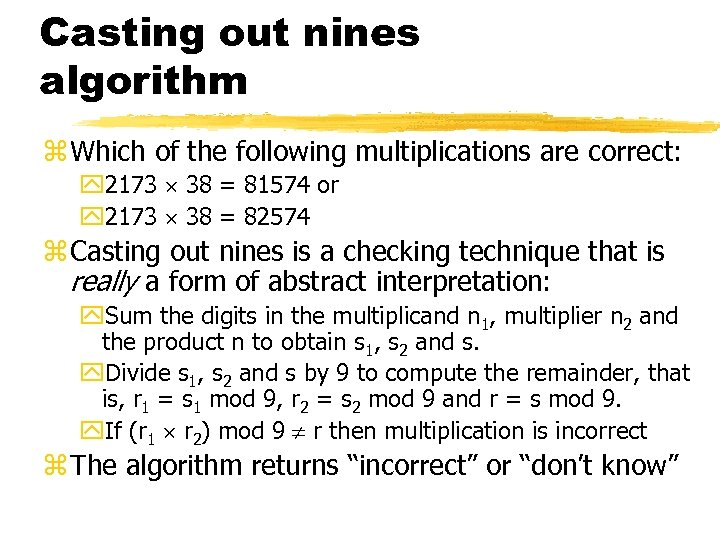Casting out nines algorithm z Which of the following multiplications are correct: y 2173 38 = 81574 or y 2173 38 = 82574 z Casting out nines is a checking technique that is really a form of abstract interpretation: y. Sum the digits in the multiplicand n 1, multiplier n 2 and the product n to obtain s 1, s 2 and s. y. Divide s 1, s 2 and s by 9 to compute the remainder, that is, r 1 = s 1 mod 9, r 2 = s 2 mod 9 and r = s mod 9. y. If (r 1 r 2) mod 9 r then multiplication is incorrect z The algorithm returns “incorrect” or “don’t know”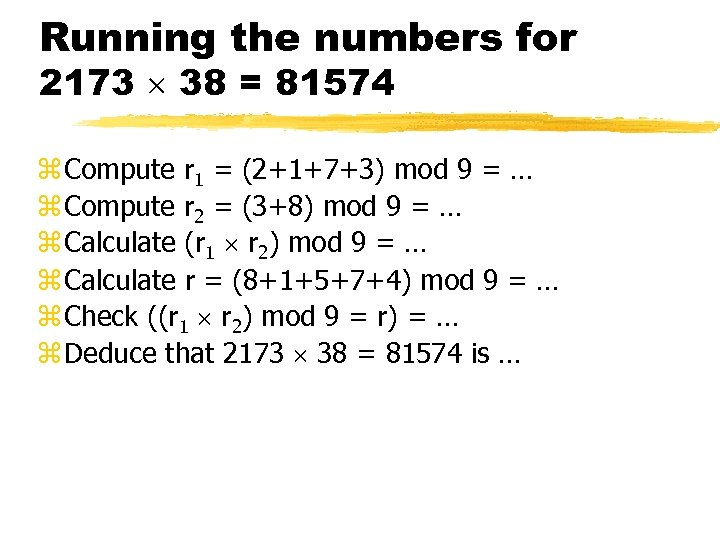Running the numbers for 2173 38 = 81574 z Compute r 1 = (2+1+7+3) mod 9 = … z Compute r 2 = (3+8) mod 9 = … z Calculate (r 1 r 2) mod 9 = … z Calculate r = (8+1+5+7+4) mod 9 = … z Check ((r 1 r 2) mod 9 = r) = … z Deduce that 2173 38 = 81574 is …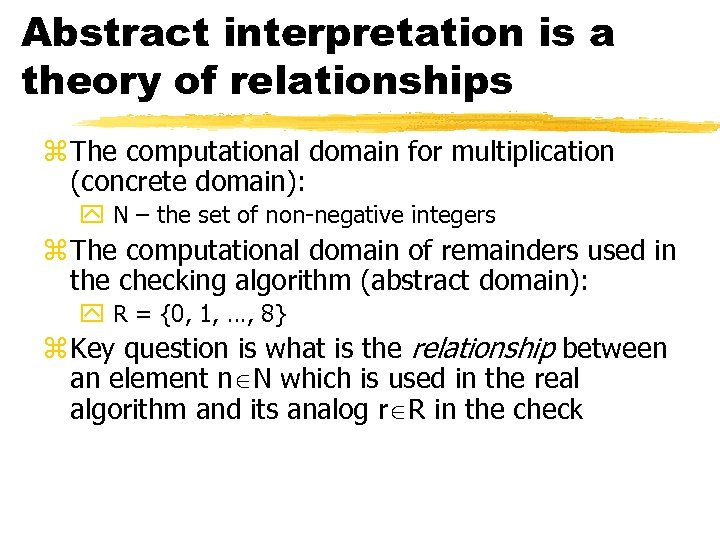Abstract interpretation is a theory of relationships z The computational domain for multiplication (concrete domain): y N – the set of non-negative integers z The computational domain of remainders used in the checking algorithm (abstract domain): y R = {0, 1, …, 8} z Key question is what is the relationship between an element n N which is used in the real algorithm and its analog r R in the check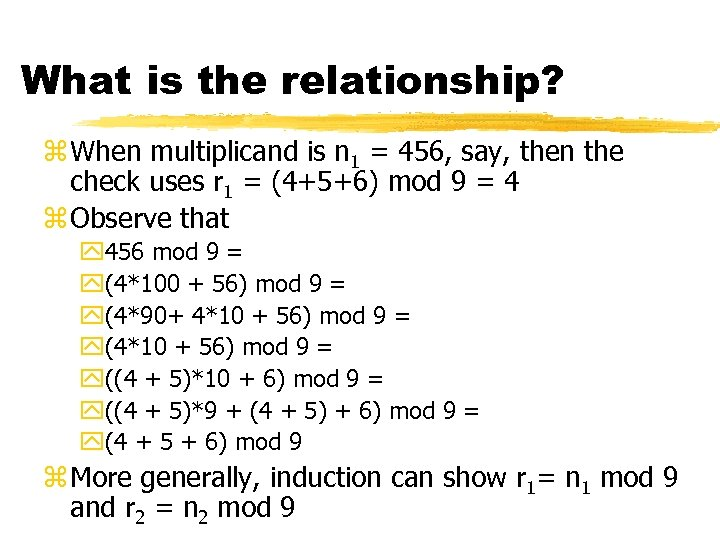What is the relationship? z When multiplicand is n 1 = 456, say, then the check uses r 1 = (4+5+6) mod 9 = 4 z Observe that y 456 mod 9 = y(4*100 + 56) mod 9 = y(4*90+ 4*10 + 56) mod 9 = y((4 + 5)*10 + 6) mod 9 = y((4 + 5)*9 + (4 + 5) + 6) mod 9 = y(4 + 5 + 6) mod 9 z More generally, induction can show r 1= n 1 mod 9 and r 2 = n 2 mod 9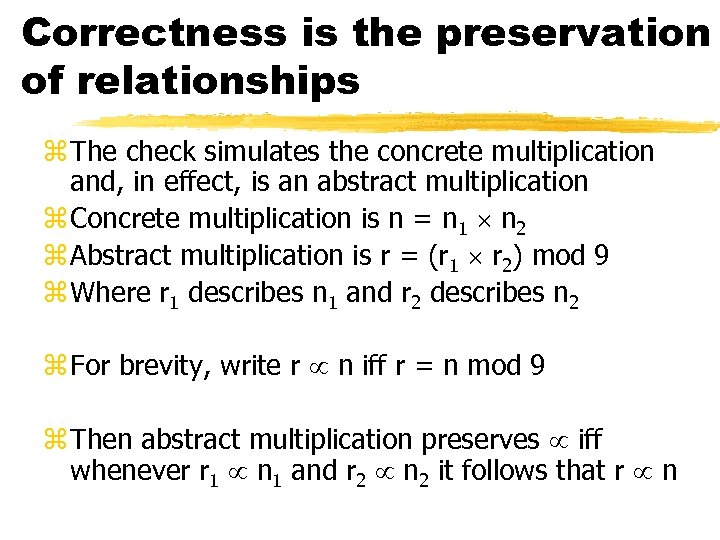Correctness is the preservation of relationships z The check simulates the concrete multiplication and, in effect, is an abstract multiplication z Concrete multiplication is n = n 1 n 2 z Abstract multiplication is r = (r 1 r 2) mod 9 z Where r 1 describes n 1 and r 2 describes n 2 z For brevity, write r n iff r = n mod 9 z Then abstract multiplication preserves iff whenever r 1 n 1 and r 2 n 2 it follows that r n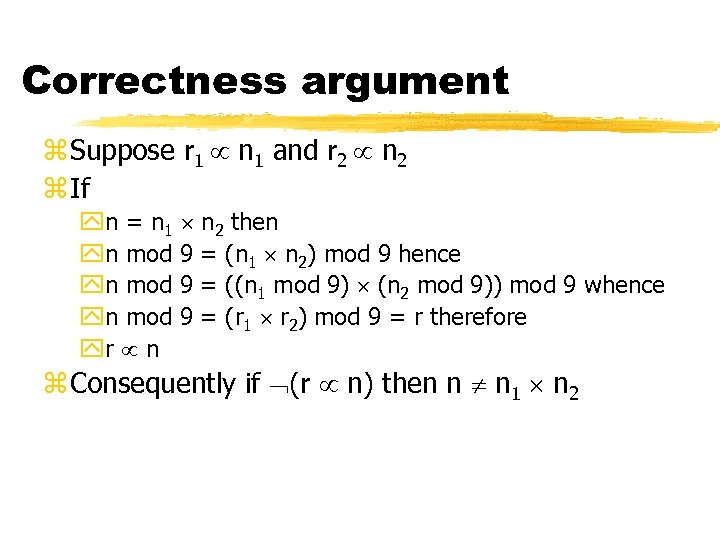Correctness argument z Suppose r 1 n 1 and r 2 n 2 z If yn = n 1 n 2 then yn mod 9 = (n 1 n 2) mod 9 hence yn mod 9 = ((n 1 mod 9) (n 2 mod 9)) mod 9 whence yn mod 9 = (r 1 r 2) mod 9 = r therefore yr n z Consequently if (r n) then n n 1 n 2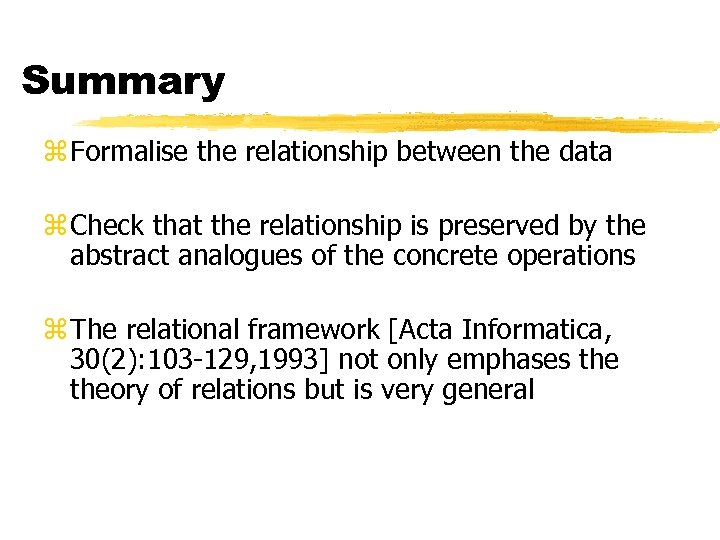Summary z Formalise the relationship between the data z Check that the relationship is preserved by the abstract analogues of the concrete operations z The relational framework [Acta Informatica, 30(2): 103 -129, 1993] not only emphases theory of relations but is very general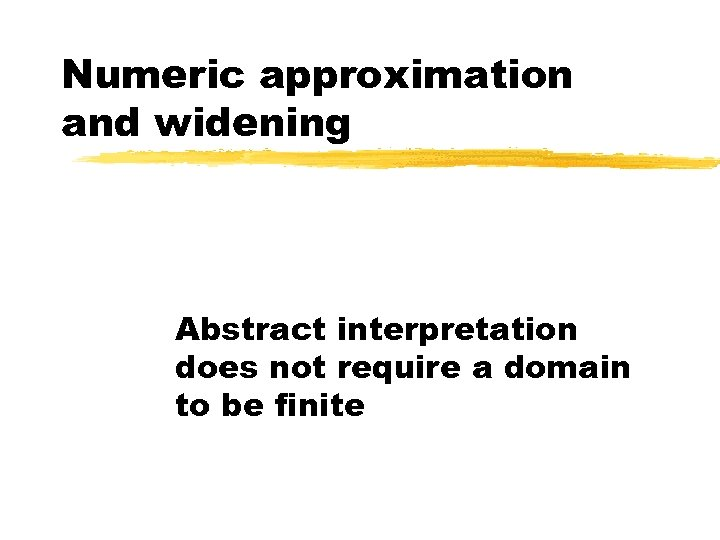Numeric approximation and widening Abstract interpretation does not require a domain to be finite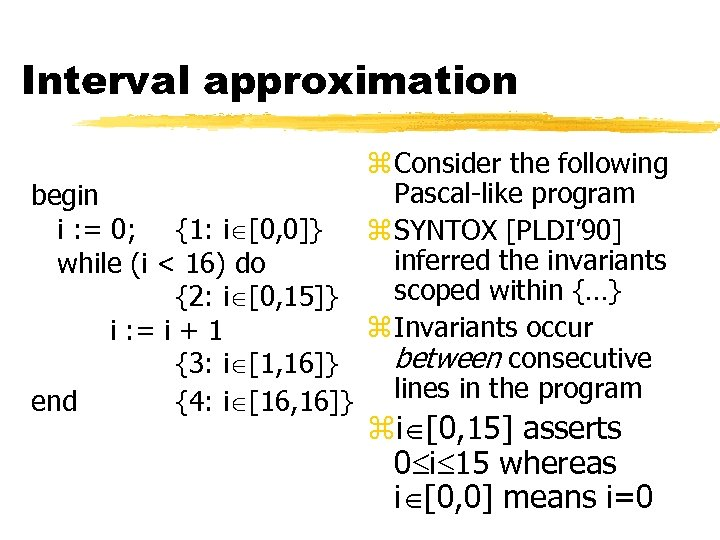Interval approximation z Consider the following Pascal-like program begin i : = 0; {1: i [0, 0]} z SYNTOX [PLDI’ 90] inferred the invariants while (i < 16) do scoped within {…} {2: i [0, 15]} z Invariants occur i : = i + 1 between consecutive {3: i [1, 16]} end {4: i [16, 16]} lines in the program zi [0, 15] asserts 0 i 15 whereas i [0, 0] means i=0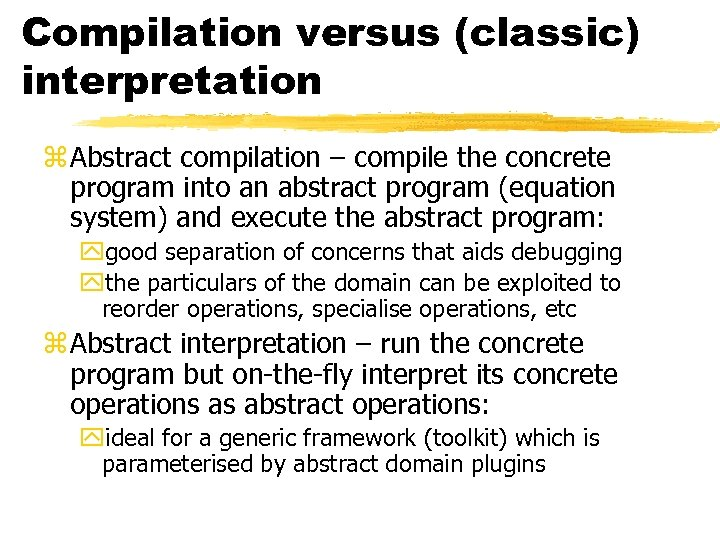Compilation versus (classic) interpretation z Abstract compilation – compile the concrete program into an abstract program (equation system) and execute the abstract program: ygood separation of concerns that aids debugging ythe particulars of the domain can be exploited to reorder operations, specialise operations, etc z Abstract interpretation – run the concrete program but on-the-fly interpret its concrete operations as abstract operations: yideal for a generic framework (toolkit) which is parameterised by abstract domain plugins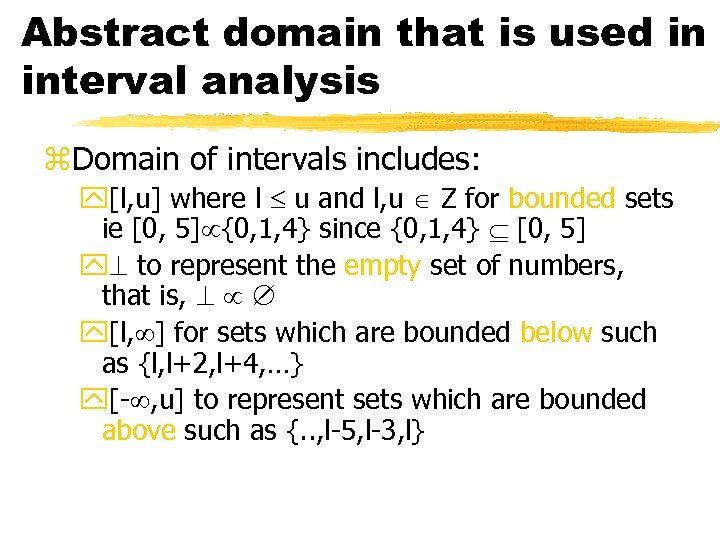Abstract domain that is used in interval analysis z. Domain of intervals includes: y[l, u] where l u and l, u Z for bounded sets ie [0, 5] {0, 1, 4} since {0, 1, 4} [0, 5] y to represent the empty set of numbers, that is, y[l, ] for sets which are bounded below such as {l, l+2, l+4, …} y[- , u] to represent sets which are bounded above such as {. . , l-5, l-3, l}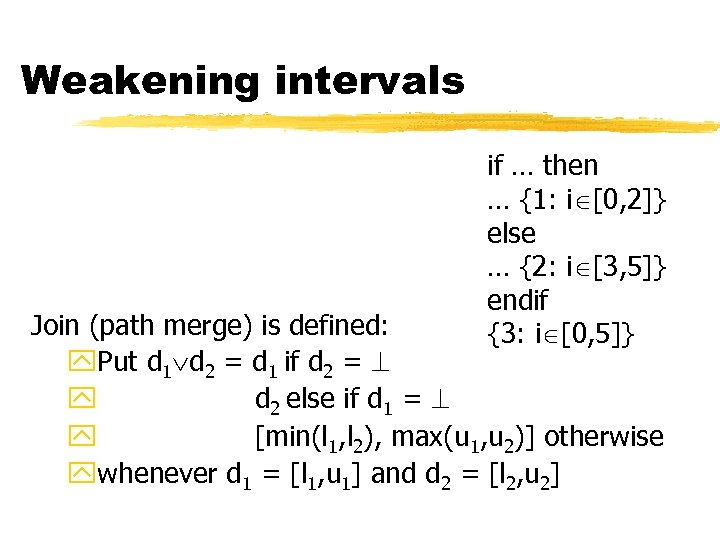Weakening intervals if … then … {1: i [0, 2]} else … {2: i [3, 5]} endif {3: i [0, 5]} Join (path merge) is defined: y. Put d 1 d 2 = d 1 if d 2 = y d 2 else if d 1 = y [min(l 1, l 2), max(u 1, u 2)] otherwise ywhenever d 1 = [l 1, u 1] and d 2 = [l 2, u 2]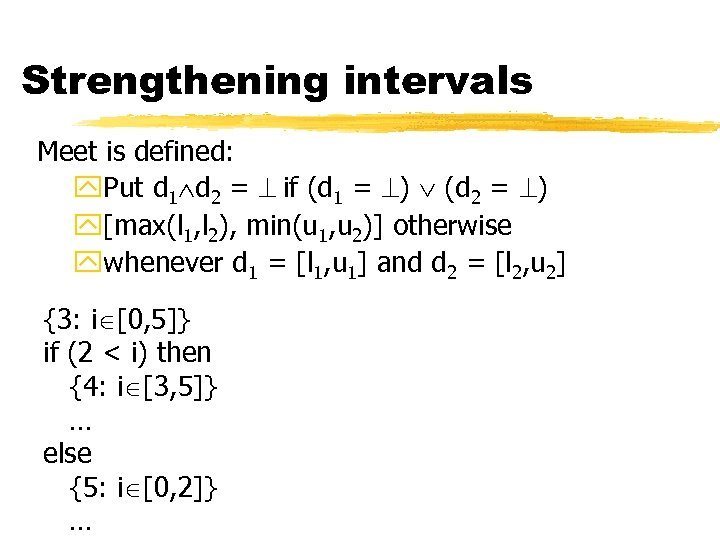Strengthening intervals Meet is defined: y. Put d 1 d 2 = if (d 1 = ) (d 2 = ) y[max(l 1, l 2), min(u 1, u 2)] otherwise ywhenever d 1 = [l 1, u 1] and d 2 = [l 2, u 2] {3: i [0, 5]} if (2 < i) then {4: i [3, 5]} … else {5: i [0, 2]} …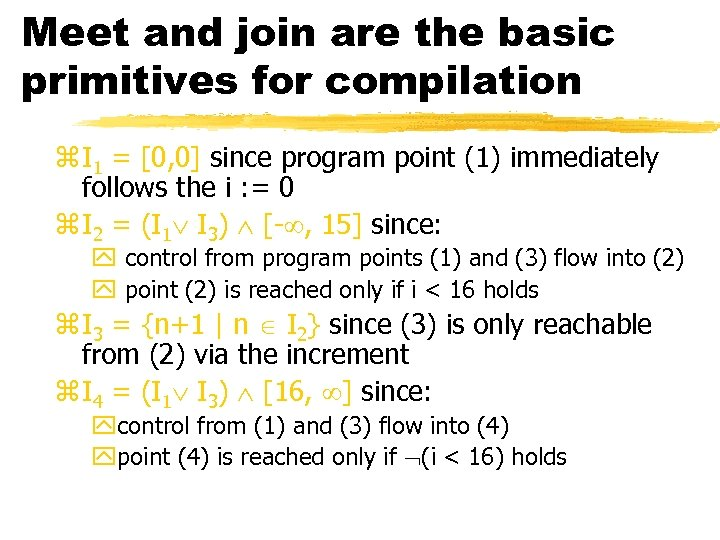Meet and join are the basic primitives for compilation z I 1 = [0, 0] since program point (1) immediately follows the i : = 0 z I 2 = (I 1 I 3) [- , 15] since: y control from program points (1) and (3) flow into (2) y point (2) is reached only if i < 16 holds z I 3 = {n+1 | n I 2} since (3) is only reachable from (2) via the increment z I 4 = (I 1 I 3) [16, ] since: ycontrol from (1) and (3) flow into (4) ypoint (4) is reached only if (i < 16) holds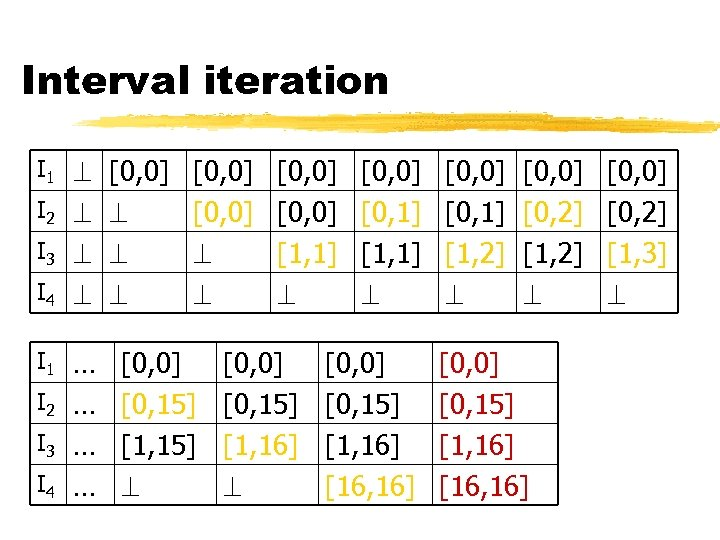Interval iteration I 1 [0, 0] [0, 0] I 2 I 3 I 4 I 1 … [0, 0] [0, 1] [0, 2] [1, 1] [1, 2] [1, 3] [0, 0] I 2 … [0, 15] I 3 … [1, 15] I 4 … [0, 0] [0, 15] [1, 16] [16, 16]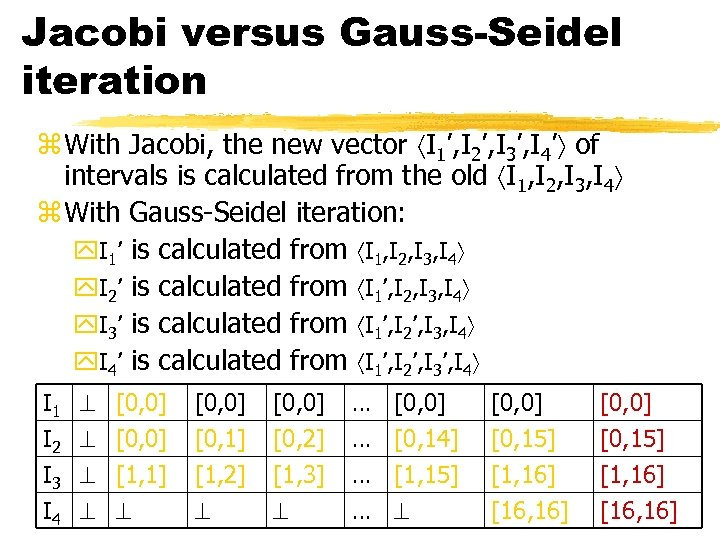Jacobi versus Gauss-Seidel iteration z With Jacobi, the new vector I 1’, I 2’, I 3’, I 4’ of intervals is calculated from the old I 1, I 2, I 3, I 4 z With Gauss-Seidel iteration: y. I 1’ is calculated from I 1, I 2, I 3, I 4 y. I 2’ is calculated from I 1’, I 2, I 3, I 4 y. I 3’ is calculated from I 1’, I 2’, I 3, I 4 y. I 4’ is calculated from I 1’, I 2’, I 3’, I 4 I 1 I 2 I 3 I 4 [0, 0] [1, 1] [0, 0] [0, 1] [1, 2] [0, 0] [0, 2] [1, 3] … … [0, 0] [0, 14] [1, 15] [0, 0] [0, 15] [1, 16] [16, 16]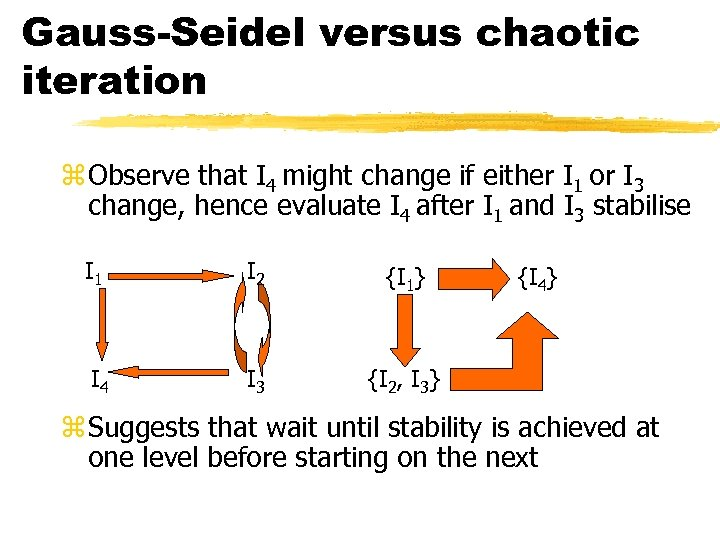Gauss-Seidel versus chaotic iteration z Observe that I 4 might change if either I 1 or I 3 change, hence evaluate I 4 after I 1 and I 3 stabilise I 1 I 2 {I 1} I 4 I 3 {I 2, I 3} {I 4} z Suggests that wait until stability is achieved at one level before starting on the next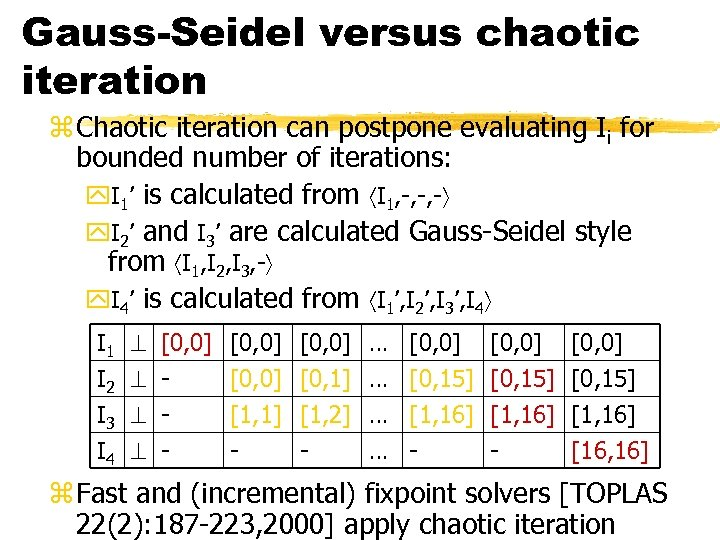Gauss-Seidel versus chaotic iteration z Chaotic iteration can postpone evaluating Ii for bounded number of iterations: y. I 1’ is calculated from I 1, -, -, - y. I 2’ and I 3’ are calculated Gauss-Seidel style from I 1, I 2, I 3, - y. I 4’ is calculated from I 1’, I 2’, I 3’, I 4 I 1 I 2 I 3 I 4 [0, 0] - [0, 0] [1, 1] - [0, 0] [0, 1] [1, 2] - … … [0, 0] [0, 15] [1, 16] - [0, 0] [0, 15] [1, 16] [16, 16] z Fast and (incremental) fixpoint solvers [TOPLAS 22(2): 187 -223, 2000] apply chaotic iteration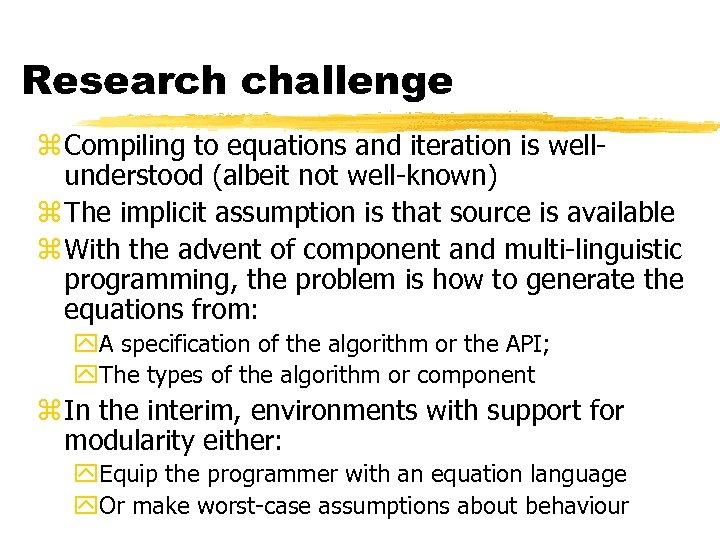Research challenge z Compiling to equations and iteration is wellunderstood (albeit not well-known) z The implicit assumption is that source is available z With the advent of component and multi-linguistic programming, the problem is how to generate the equations from: y. A specification of the algorithm or the API; y. The types of the algorithm or component z In the interim, environments with support for modularity either: y. Equip the programmer with an equation language y. Or make worst-case assumptions about behaviour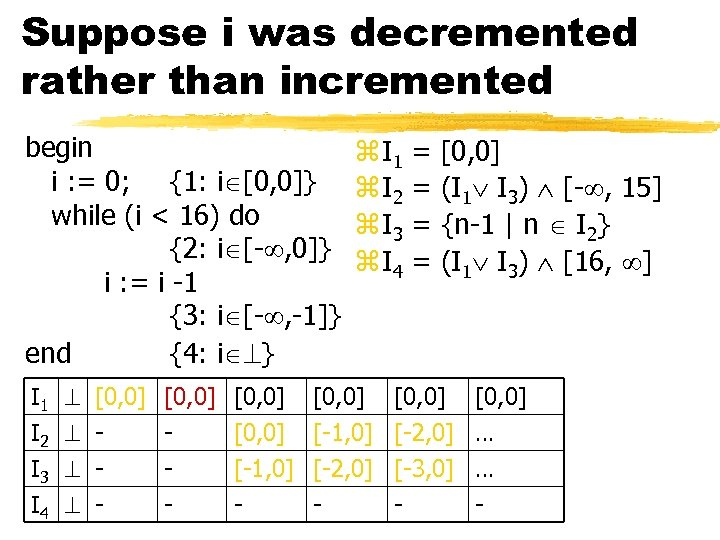Suppose i was decremented rather than incremented begin i : = 0; {1: i [0, 0]} while (i < 16) do {2: i [- , 0]} i : = i -1 {3: i [- , -1]} end {4: i } I 1 I 2 I 3 I 4 [0, 0] - [0, 0] [-1, 0] - z I 1 z I 2 z I 3 z I 4 [0, 0] [-1, 0] [-2, 0] - = = [0, 0] (I 1 I 3) [- , 15] {n-1 | n I 2} (I 1 I 3) [16, ] [0, 0] [-2, 0] [-3, 0] - [0, 0] … … -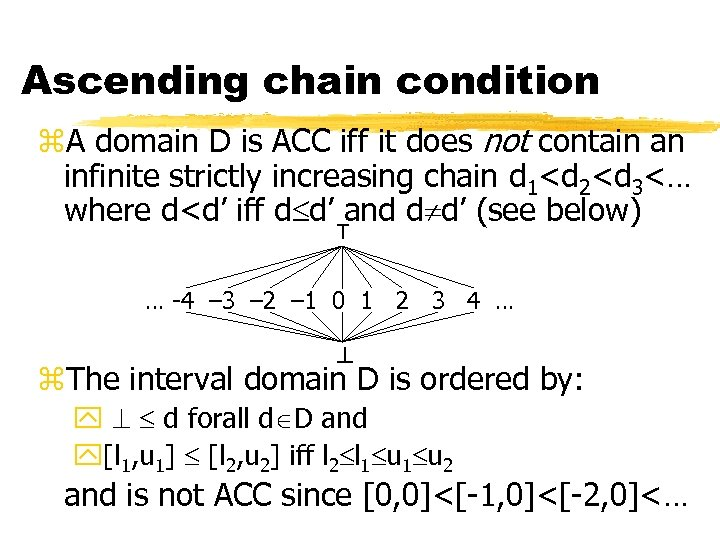Ascending chain condition z. A domain D is ACC iff it does not contain an infinite strictly increasing chain d 1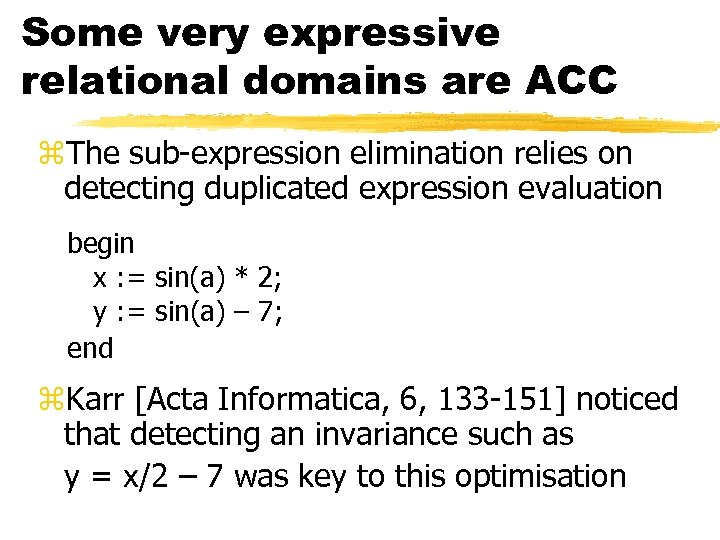Some very expressive relational domains are ACC z. The sub-expression elimination relies on detecting duplicated expression evaluation begin x : = sin(a) * 2; y : = sin(a) – 7; end z. Karr [Acta Informatica, 6, 133 -151] noticed that detecting an invariance such as y = x/2 – 7 was key to this optimisation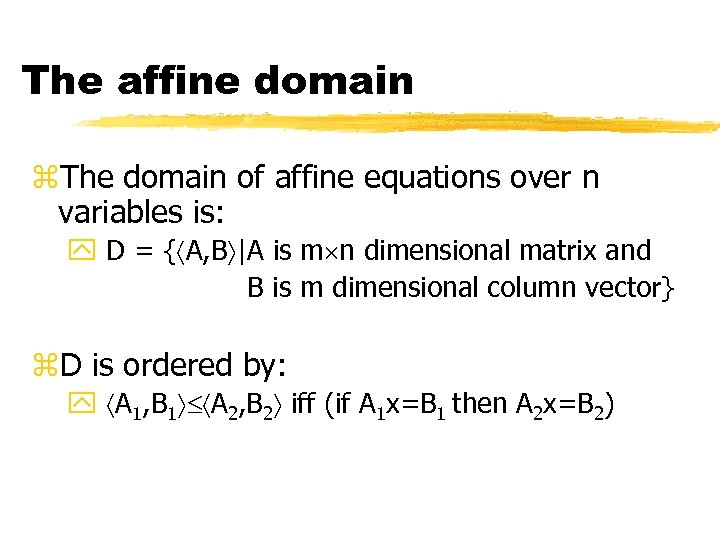The affine domain z. The domain of affine equations over n variables is: y D = { A, B |A is m n dimensional matrix and B is m dimensional column vector} z. D is ordered by: y A 1, B 1 A 2, B 2 iff (if A 1 x=B 1 then A 2 x=B 2)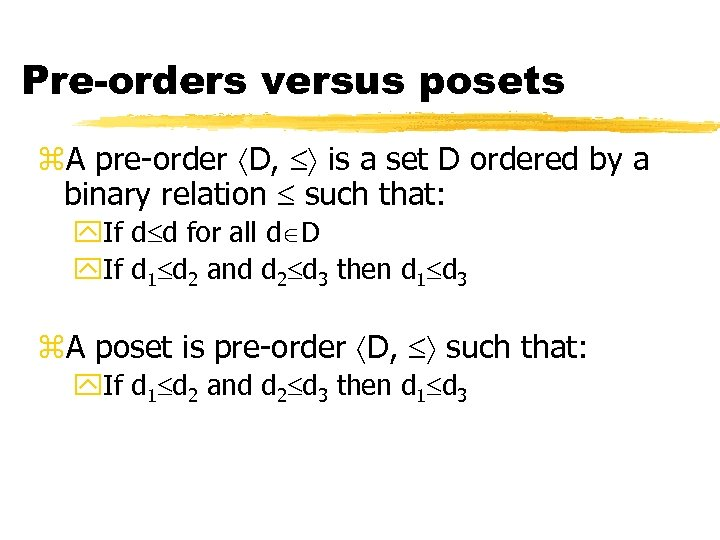Pre-orders versus posets z. A pre-order D, is a set D ordered by a binary relation such that: y. If d d for all d D y. If d 1 d 2 and d 2 d 3 then d 1 d 3 z. A poset is pre-order D, such that: y. If d 1 d 2 and d 2 d 3 then d 1 d 3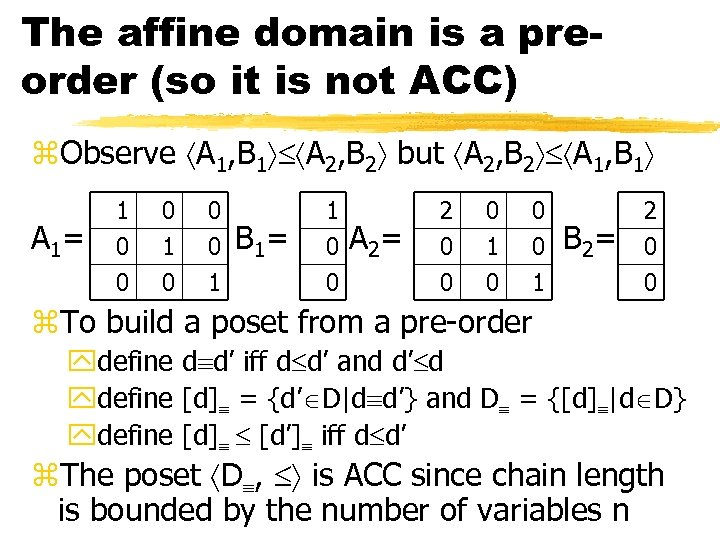The affine domain is a preorder (so it is not ACC) z. Observe A 1, B 1 A 2, B 2 but A 2, B 2 A 1, B 1 A 1= 1 0 0 0 1 B 1= 1 0 0 A 2= 2 0 0 0 1 B 2= 2 0 0 z. To build a poset from a pre-order ydefine d d’ iff d d’ and d’ d ydefine [d] = {d’ D|d d’} and D = {[d] |d D} ydefine [d] [d’] iff d d’ z. The poset D , is ACC since chain length is bounded by the number of variables n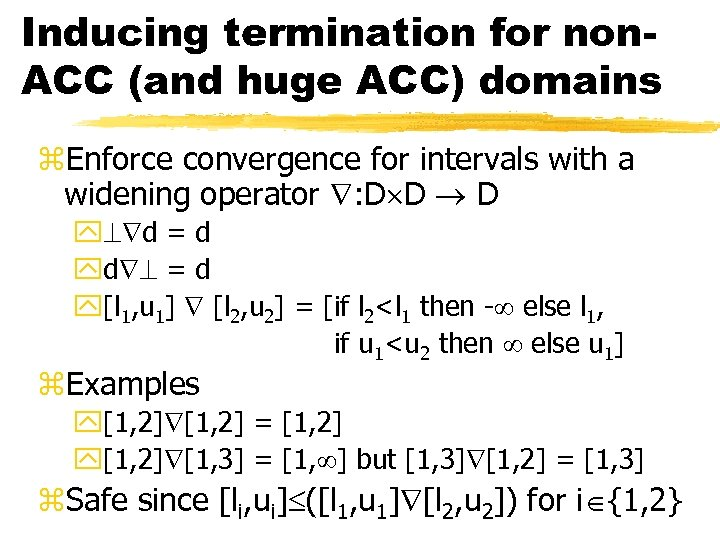Inducing termination for non. ACC (and huge ACC) domains z. Enforce convergence for intervals with a widening operator : D D D y d = d yd = d y[l 1, u 1] [l 2, u 2] = [if l 2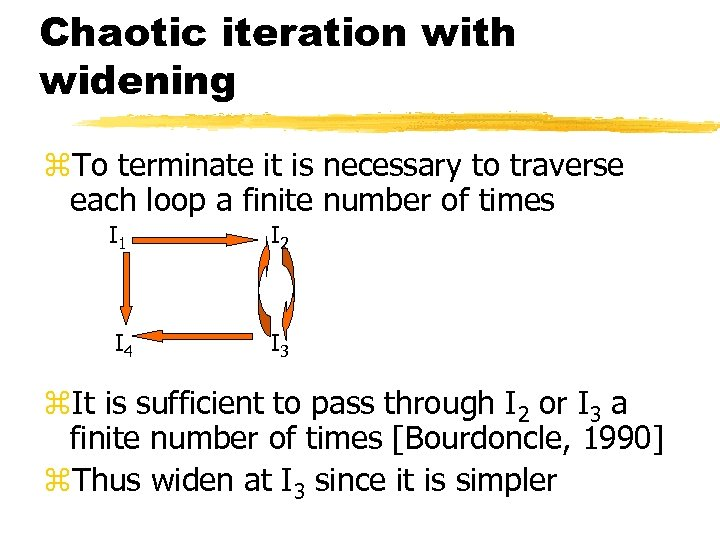Chaotic iteration with widening z. To terminate it is necessary to traverse each loop a finite number of times I 1 I 2 I 4 I 3 z. It is sufficient to pass through I 2 or I 3 a finite number of times [Bourdoncle, 1990] z. Thus widen at I 3 since it is simpler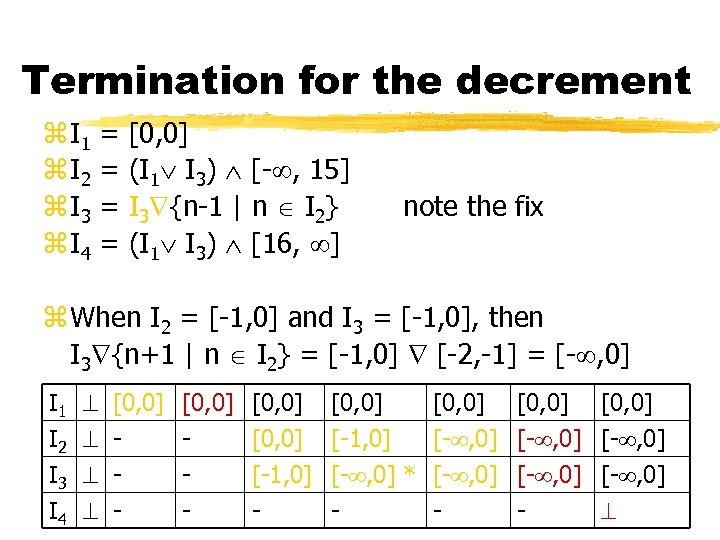Termination for the decrement z I 1 z I 2 z I 3 z I 4 = = [0, 0] (I 1 I 3) [- , 15] I 3 {n-1 | n I 2} (I 1 I 3) [16, ] note the fix z When I 2 = [-1, 0] and I 3 = [-1, 0], then I 3 {n+1 | n I 2} = [-1, 0] [-2, -1] = [- , 0] I 1 I 2 I 3 I 4 [0, 0] - [0, 0] [-1, 0] - [0, 0] [- , 0] [-1, 0] [- , 0] * [- , 0] -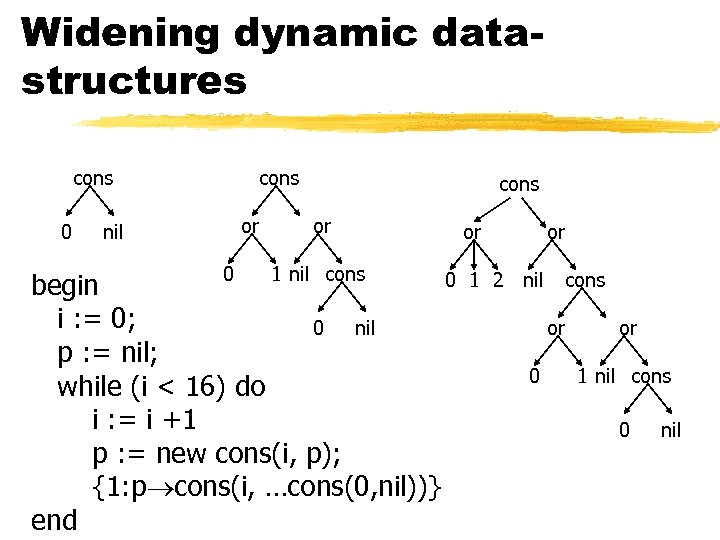Widening dynamic datastructures cons 0 nil cons or 0 1 nil cons 0 begin i : = 0; 0 nil p : = nil; while (i < 16) do i : = i +1 p : = new cons(i, p); {1: p cons(i, …cons(0, nil))} end or or 1 2 nil cons or 0 or 1 nil cons 0 nil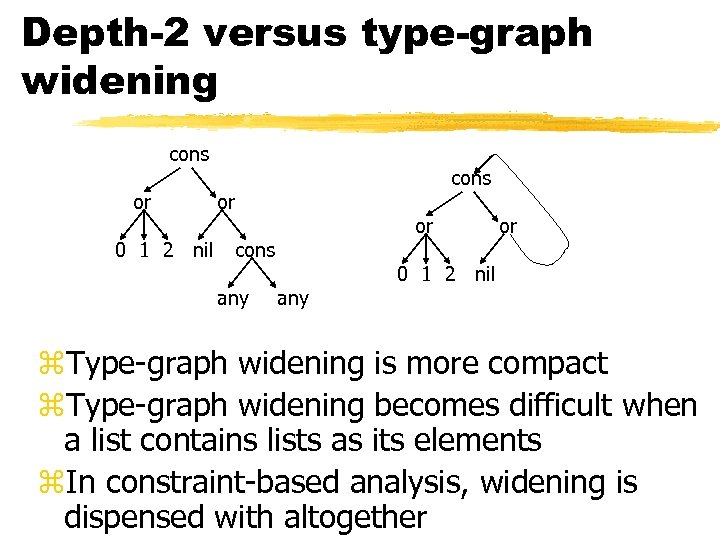Depth-2 versus type-graph widening cons or 0 1 2 nil cons or or cons any or 0 1 2 nil z. Type-graph widening is more compact z. Type-graph widening becomes difficult when a list contains lists as its elements z. In constraint-based analysis, widening is dispensed with altogether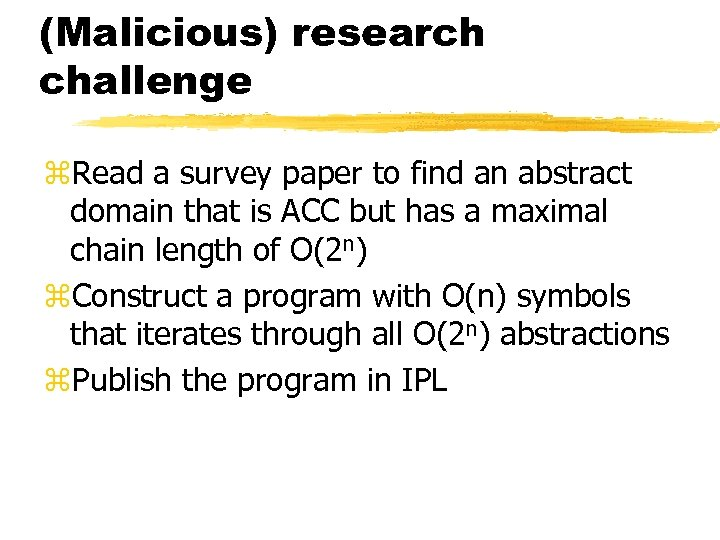(Malicious) research challenge z. Read a survey paper to find an abstract domain that is ACC but has a maximal chain length of O(2 n) z. Construct a program with O(n) symbols that iterates through all O(2 n) abstractions z. Publish the program in IPL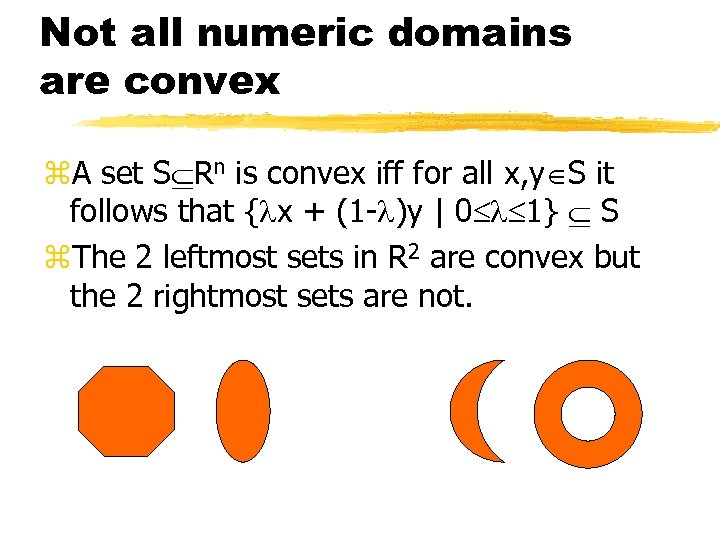Not all numeric domains are convex z. A set S Rn is convex iff for all x, y S it follows that { x + (1 - )y | 0 1} S z. The 2 leftmost sets in R 2 are convex but the 2 rightmost sets are not.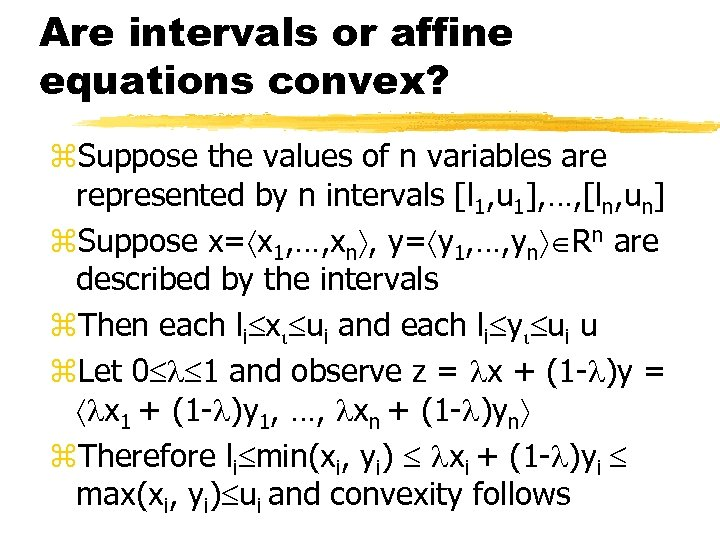Are intervals or affine equations convex? z. Suppose the values of n variables are represented by n intervals [l 1, u 1], …, [ln, un] z. Suppose x= x 1, …, xn , y= y 1, …, yn Rn are described by the intervals z. Then each li xi ui and each li yi ui u z. Let 0 1 and observe z = x + (1 - )y = x 1 + (1 - )y 1, …, xn + (1 - )yn z. Therefore li min(xi, yi) xi + (1 - )yi max(xi, yi) ui and convexity follows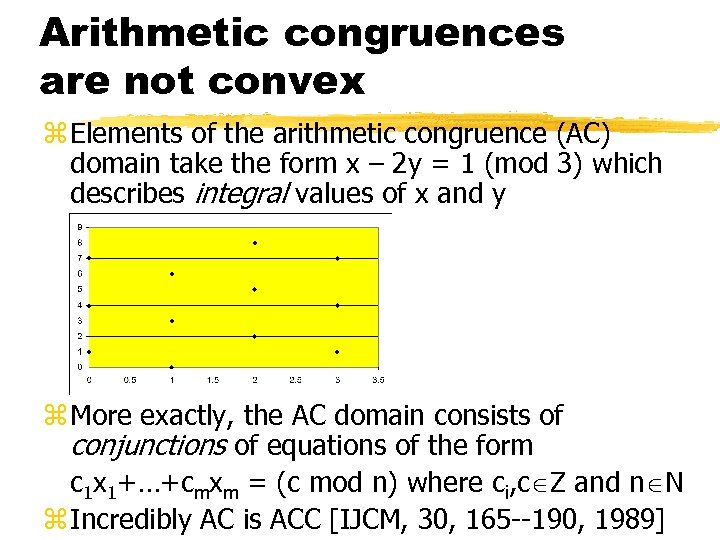Arithmetic congruences are not convex z Elements of the arithmetic congruence (AC) domain take the form x – 2 y = 1 (mod 3) which describes integral values of x and y z More exactly, the AC domain consists of conjunctions of equations of the form c 1 x 1+…+cmxm = (c mod n) where ci, c Z and n N z Incredibly AC is ACC [IJCM, 30, 165 --190, 1989]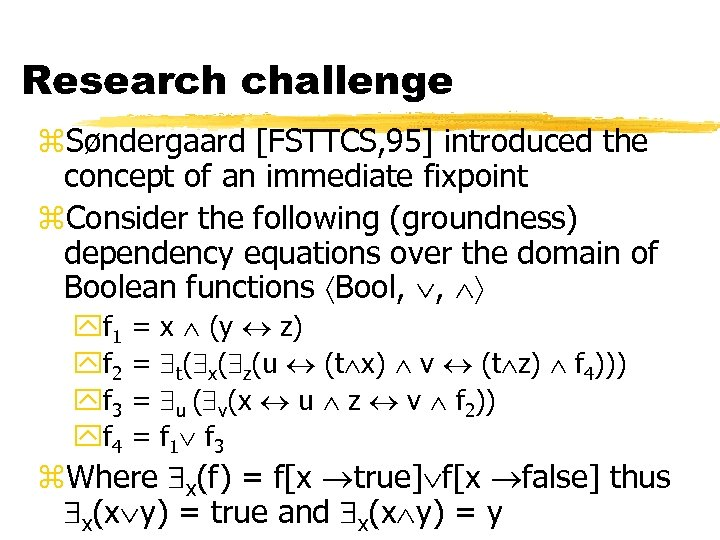Research challenge z. Søndergaard [FSTTCS, 95] introduced the concept of an immediate fixpoint z. Consider the following (groundness) dependency equations over the domain of Boolean functions Bool, , yf 1 yf 2 yf 3 yf 4 = = x (y z) t( x( z(u (t x) v (t z) f 4))) u ( v(x u z v f 2)) f 1 f 3 z. Where x(f) = f[x true] f[x false] thus x(x y) = true and x(x y) = y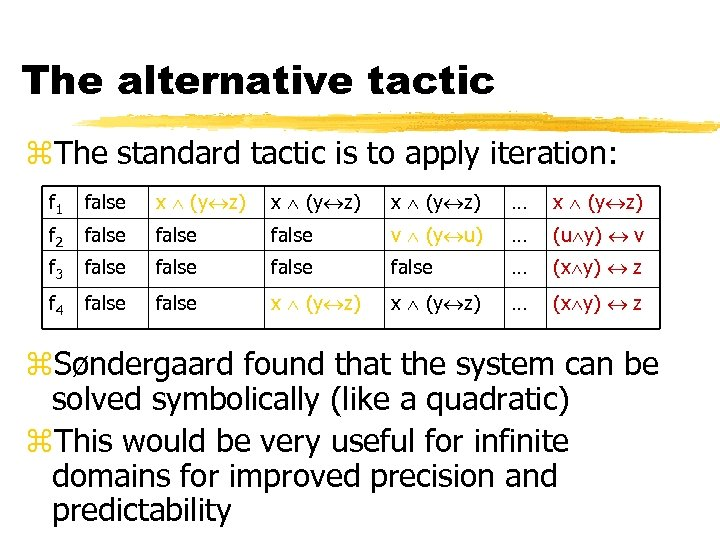The alternative tactic z. The standard tactic is to apply iteration: f 1 false x (y z) … x (y z) f 2 false v (y u) … (u y) v f 3 false … (x y) z f 4 false x (y z) … (x y) z z. Søndergaard found that the system can be solved symbolically (like a quadratic) z. This would be very useful for infinite domains for improved precision and predictability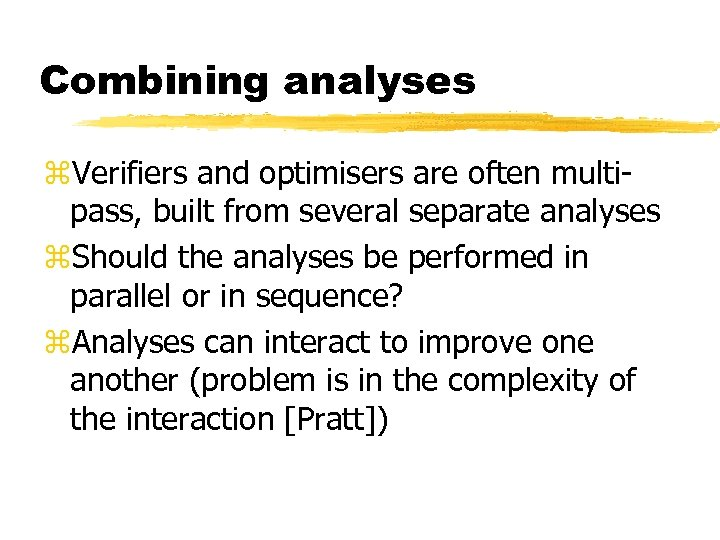Combining analyses z. Verifiers and optimisers are often multipass, built from several separate analyses z. Should the analyses be performed in parallel or in sequence? z. Analyses can interact to improve one another (problem is in the complexity of the interaction [Pratt])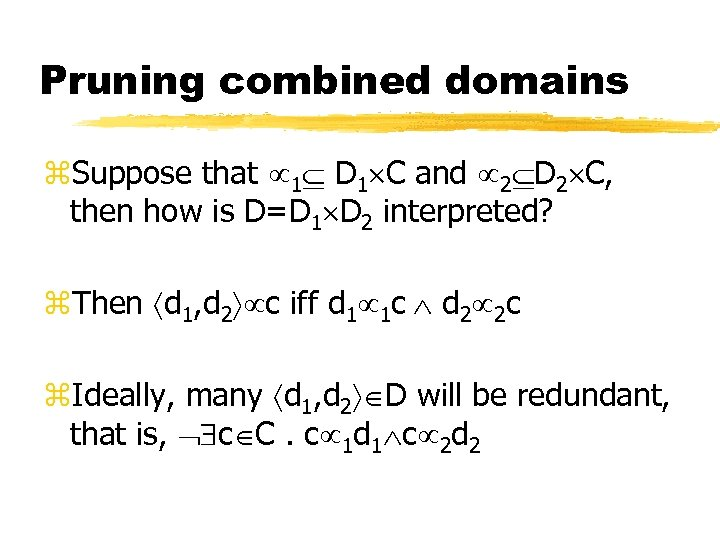Pruning combined domains z. Suppose that 1 D 1 C and 2 D 2 C, then how is D=D 1 D 2 interpreted? z. Then d 1, d 2 c iff d 1 1 c d 2 2 c z. Ideally, many d 1, d 2 D will be redundant, that is, c C. c 1 d 1 c 2 d 2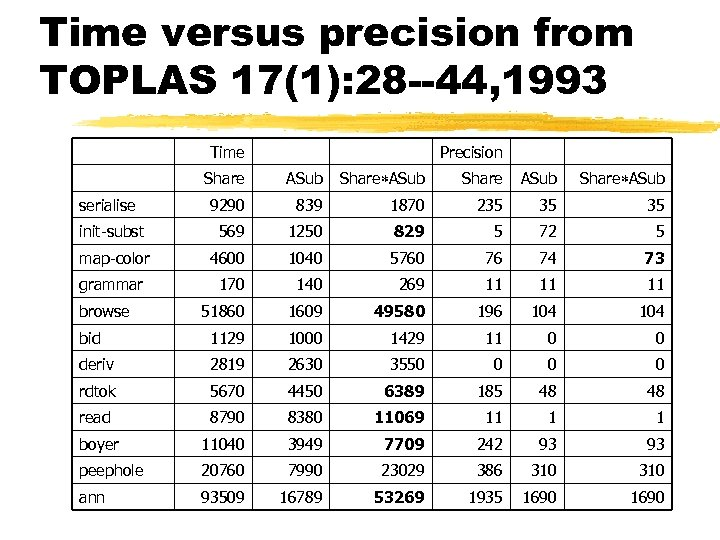Time versus precision from TOPLAS 17(1): 28 --44, 1993 Time Precision Share ASub serialise 9290 839 1870 235 35 35 init-subst 569 1250 829 5 72 5 map-color 4600 1040 5760 76 74 73 grammar 170 140 269 11 11 11 51860 1609 49580 196 104 bid 1129 1000 1429 11 0 0 deriv 2819 2630 3550 0 rdtok 5670 4450 6389 185 48 48 read 8790 8380 11069 11 1 1 boyer 11040 3949 7709 242 93 93 peephole 20760 7990 23029 386 310 ann 93509 16789 53269 1935 1690 browse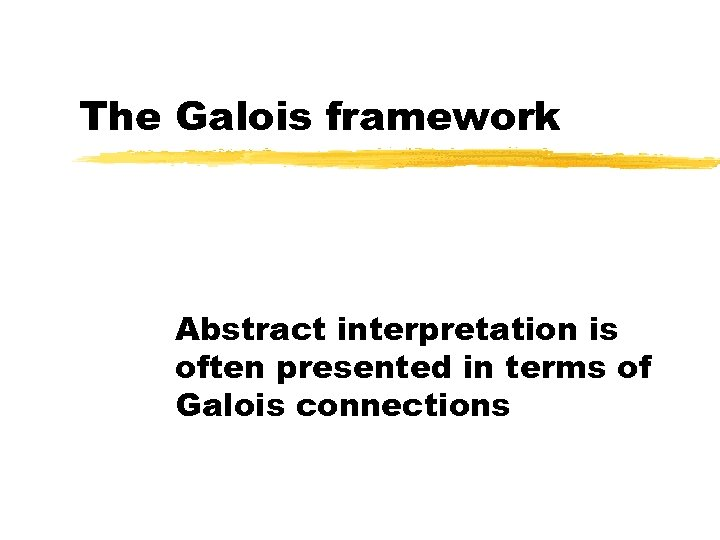The Galois framework Abstract interpretation is often presented in terms of Galois connections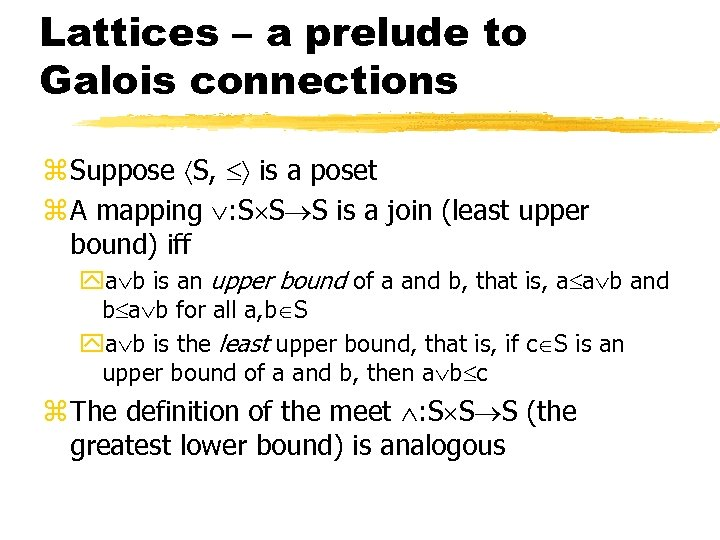Lattices – a prelude to Galois connections z Suppose S, is a poset z A mapping : S S S is a join (least upper bound) iff ya b is an upper bound of a and b, that is, a a b and b a b for all a, b S ya b is the least upper bound, that is, if c S is an upper bound of a and b, then a b c z The definition of the meet : S S S (the greatest lower bound) is analogousComplete lattices z A lattice S, , , is a poset S, equipped with a join and a meet z The join concept can often be lifted to sets by defining : (S) S iff yt ( T) for all T S and for all t T yif t s for all t T then ( T) s z If meet can often be lifted analogously, then the lattice is complete z A lattice that contains a finite number of elements is always complete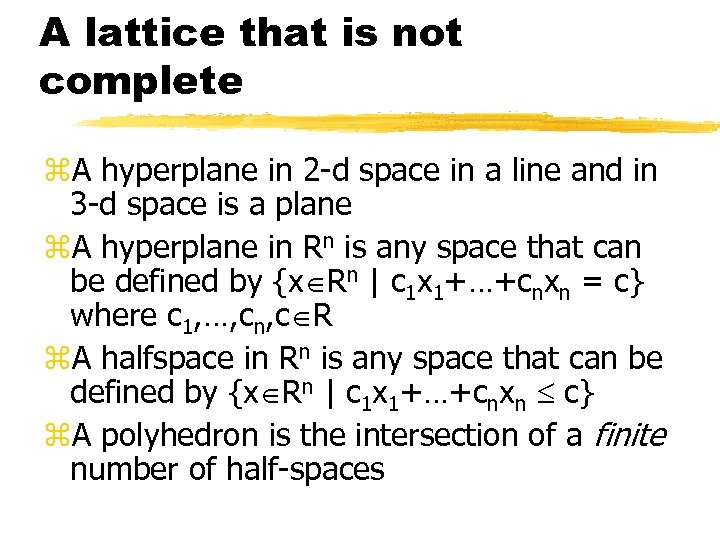A lattice that is not complete z. A hyperplane in 2 -d space in a line and in 3 -d space is a plane z. A hyperplane in Rn is any space that can be defined by {x Rn | c 1 x 1+…+cnxn = c} where c 1, …, cn, c R z. A halfspace in Rn is any space that can be defined by {x Rn | c 1 x 1+…+cnxn c} z. A polyhedron is the intersection of a finite number of half-spaces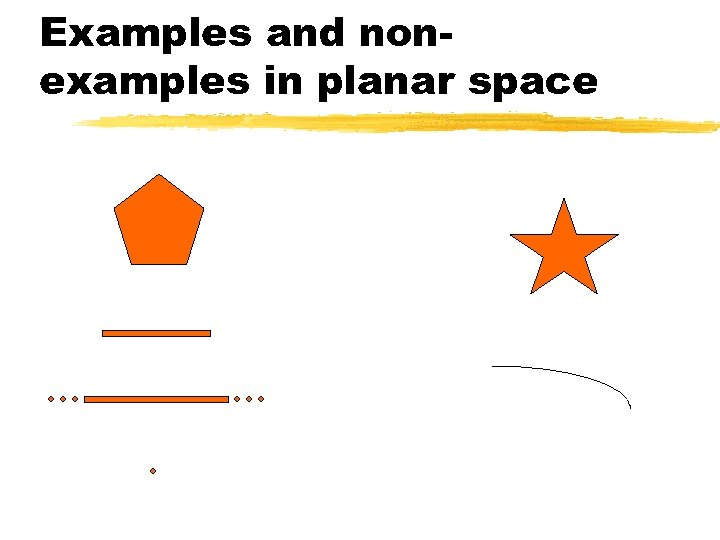Examples and nonexamples in planar space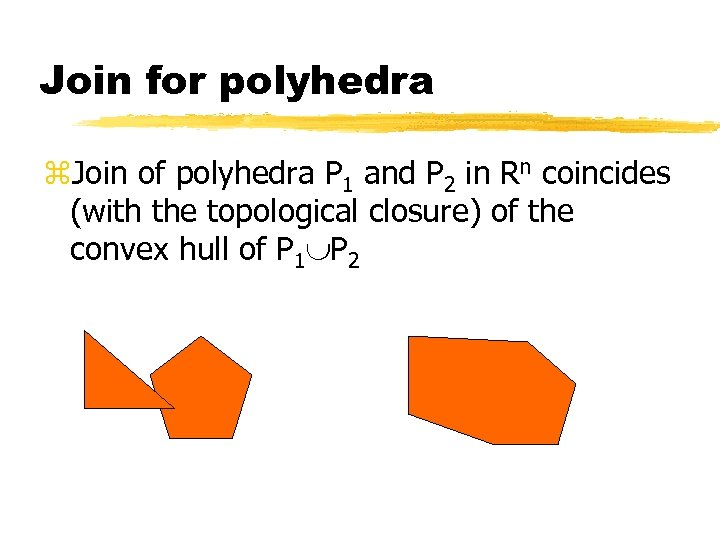Join for polyhedra z. Join of polyhedra P 1 and P 2 in Rn coincides (with the topological closure) of the convex hull of P 1 P 2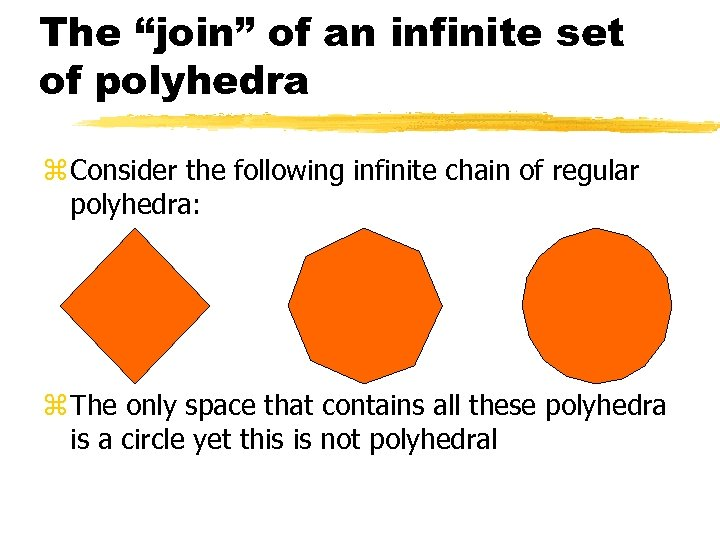The “join” of an infinite set of polyhedra z Consider the following infinite chain of regular polyhedra: z The only space that contains all these polyhedra is a circle yet this is not polyhedral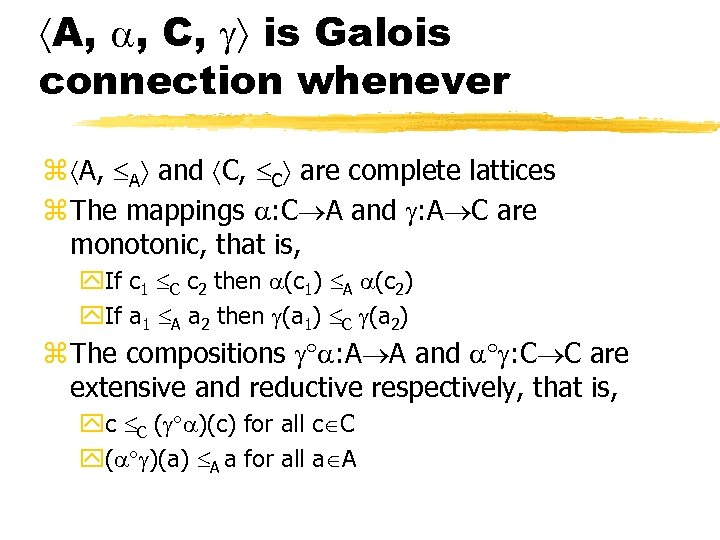A, , C, is Galois connection whenever z A, A and C, C are complete lattices z The mappings : C A and : A C are monotonic, that is, y. If c 1 C c 2 then (c 1) A (c 2) y. If a 1 A a 2 then (a 1) C (a 2) z The compositions : A A and : C C are extensive and reductive respectively, that is, yc C ( )(c) for all c C y( )(a) A a for all a A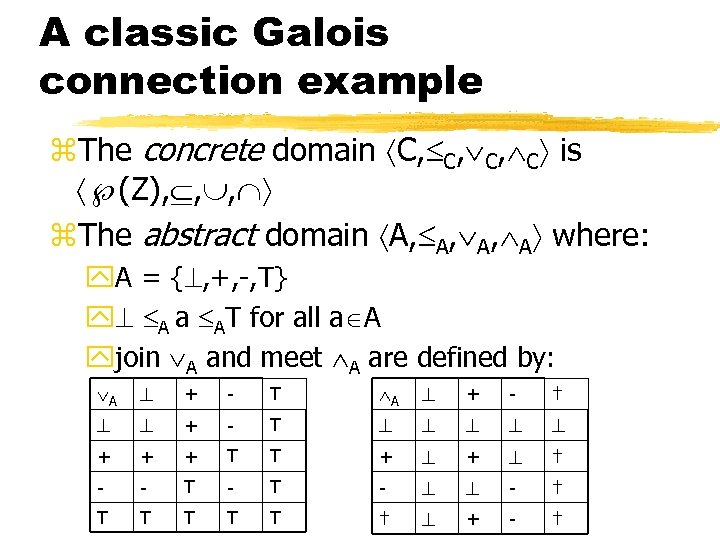A classic Galois connection example z. The concrete domain C, C, C, C is (Z), , , z. The abstract domain A, A, A, A where: y. A = { , +, -, T} y A a AT for all a A yjoin A and meet A are defined by: A + - T A + - † + - T + + + T T + + † - - T - - † T T T † + - †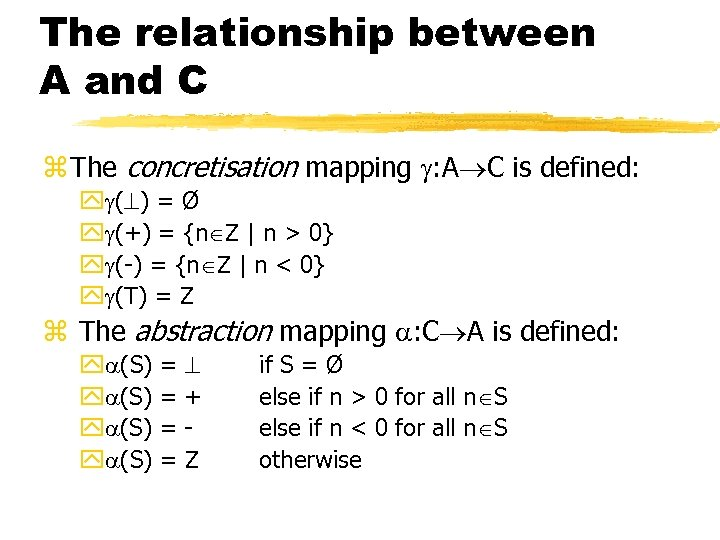The relationship between A and C z The concretisation mapping : A C is defined: y ( ) = Ø y (+) = {n Z | n > 0} y (-) = {n Z | n < 0} y (T) = Z z The abstraction mapping : C A is defined: y (S) = = + Z if S = Ø else if n > 0 for all n S else if n < 0 for all n S otherwise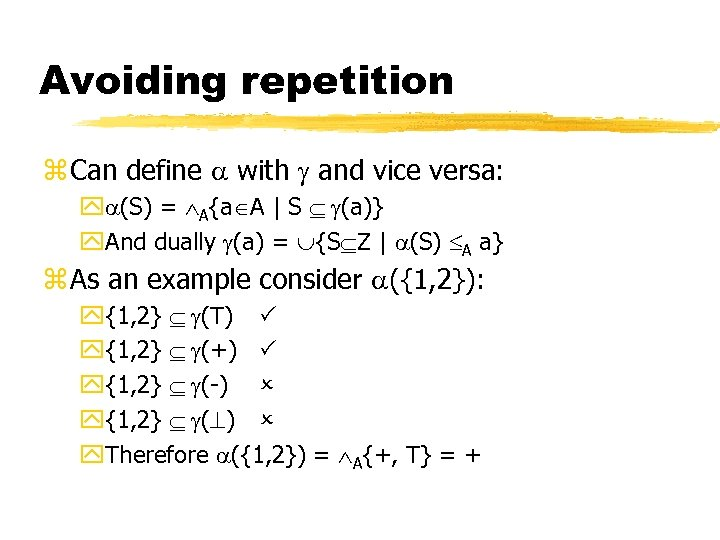Avoiding repetition z Can define with and vice versa: y (S) = A{a A | S (a)} y. And dually (a) = {S Z | (S) A a} z As an example consider ({1, 2}): y{1, 2} (T) y{1, 2} (+) y{1, 2} (-) y{1, 2} ( ) y. Therefore ({1, 2}) = A{+, T} = +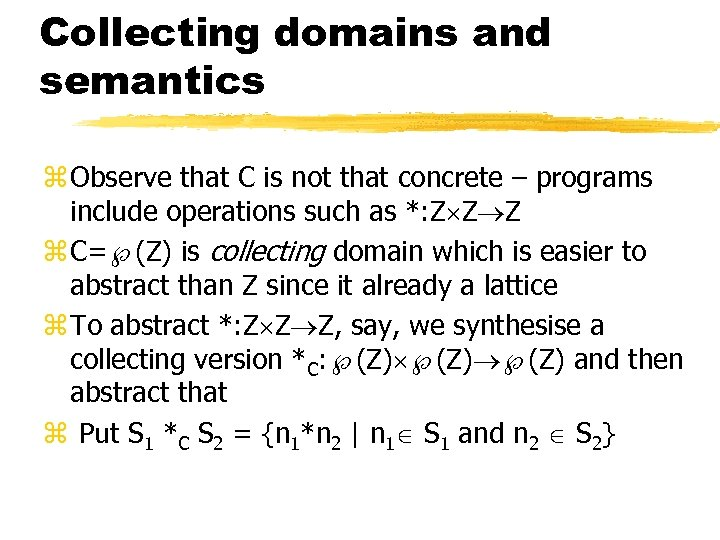Collecting domains and semantics z Observe that C is not that concrete – programs include operations such as *: Z Z Z z C= (Z) is collecting domain which is easier to abstract than Z since it already a lattice z To abstract *: Z Z Z, say, we synthesise a collecting version *C: (Z) (Z) and then abstract that z Put S 1 *C S 2 = {n 1*n 2 | n 1 S 1 and n 2 S 2}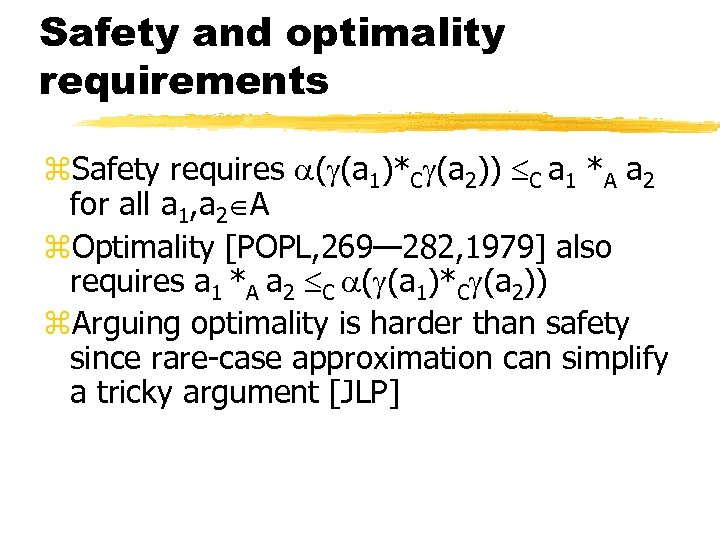Safety and optimality requirements z. Safety requires ( (a 1)*C (a 2)) C a 1 *A a 2 for all a 1, a 2 A z. Optimality [POPL, 269— 282, 1979] also requires a 1 *A a 2 C ( (a 1)*C (a 2)) z. Arguing optimality is harder than safety since rare-case approximation can simplify a tricky argument [JLP]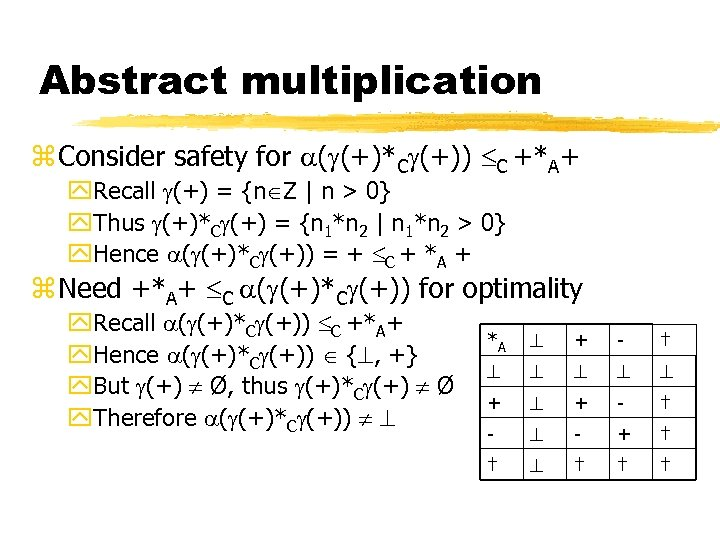Abstract multiplication z Consider safety for ( (+)*C (+)) C +*A+ y. Recall (+) = {n Z | n > 0} y. Thus (+)*C (+) = {n 1*n 2 | n 1*n 2 > 0} y. Hence ( (+)*C (+)) = + C + *A + z Need +*A+ C ( (+)*C (+)) for optimality y. Recall ( (+)*C (+)) C +*A+ y. Hence ( (+)*C (+)) { , +} y. But (+) Ø, thus (+)*C (+) Ø y. Therefore ( (+)*C (+)) *A + - † + + - † - - + † † †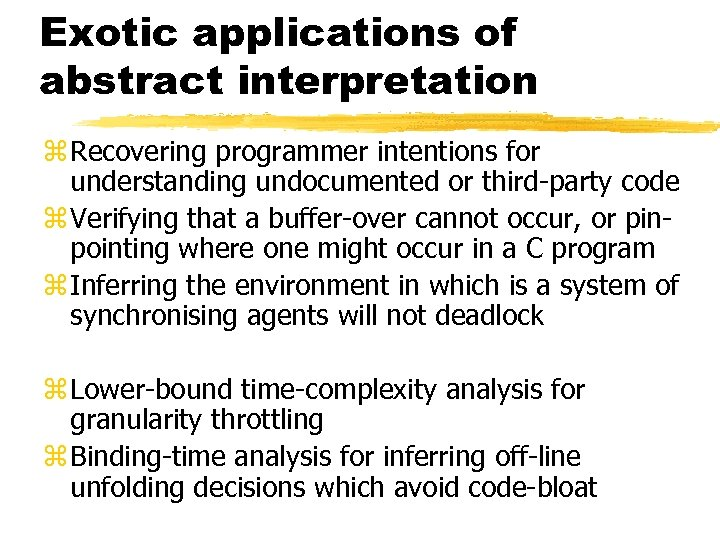Exotic applications of abstract interpretation z Recovering programmer intentions for understanding undocumented or third-party code z Verifying that a buffer-over cannot occur, or pinpointing where one might occur in a C program z Inferring the environment in which is a system of synchronising agents will not deadlock z Lower-bound time-complexity analysis for granularity throttling z Binding-time analysis for inferring off-line unfolding decisions which avoid code-bloat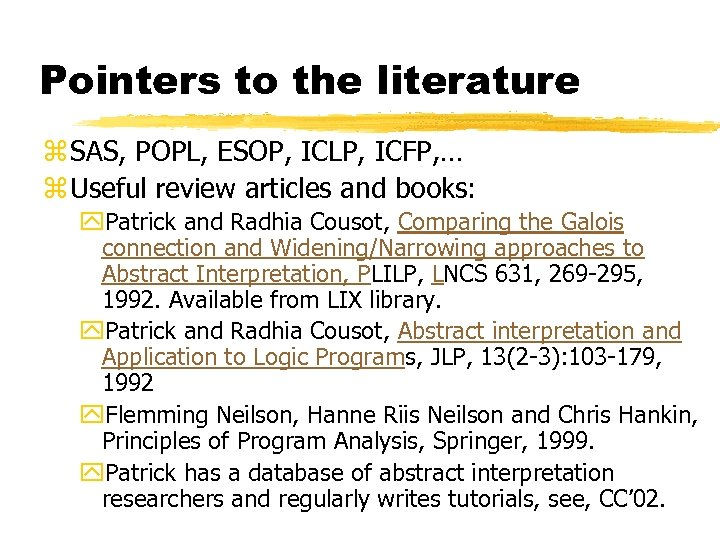Pointers to the literature z SAS, POPL, ESOP, ICLP, ICFP, … z Useful review articles and books: y. Patrick and Radhia Cousot, Comparing the Galois connection and Widening/Narrowing approaches to Abstract Interpretation, PLILP, LNCS 631, 269 -295, 1992. Available from LIX library. y. Patrick and Radhia Cousot, Abstract interpretation and Application to Logic Programs, JLP, 13(2 -3): 103 -179, 1992 y. Flemming Neilson, Hanne Riis Neilson and Chris Hankin, Principles of Program Analysis, Springer, 1999. y. Patrick has a database of abstract interpretation researchers and regularly writes tutorials, see, CC’ 02.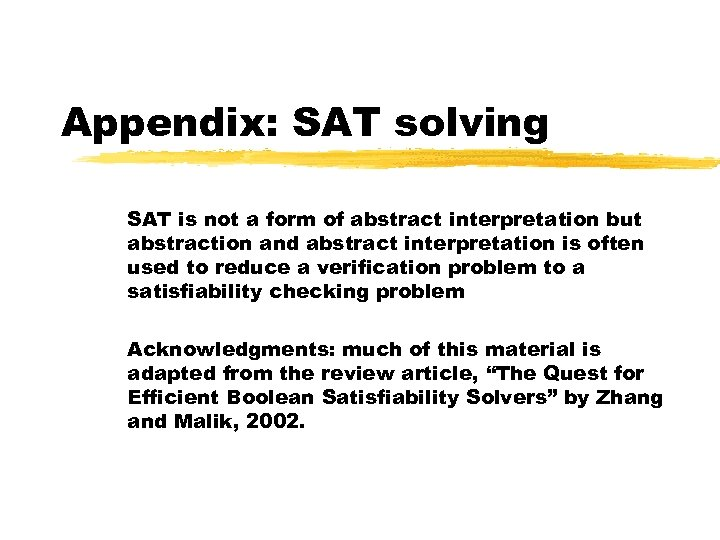Appendix: SAT solving SAT is not a form of abstract interpretation but abstraction and abstract interpretation is often used to reduce a verification problem to a satisfiability checking problem Acknowledgments: much of this material is adapted from the review article, “The Quest for Efficient Boolean Satisfiability Solvers” by Zhang and Malik, 2002.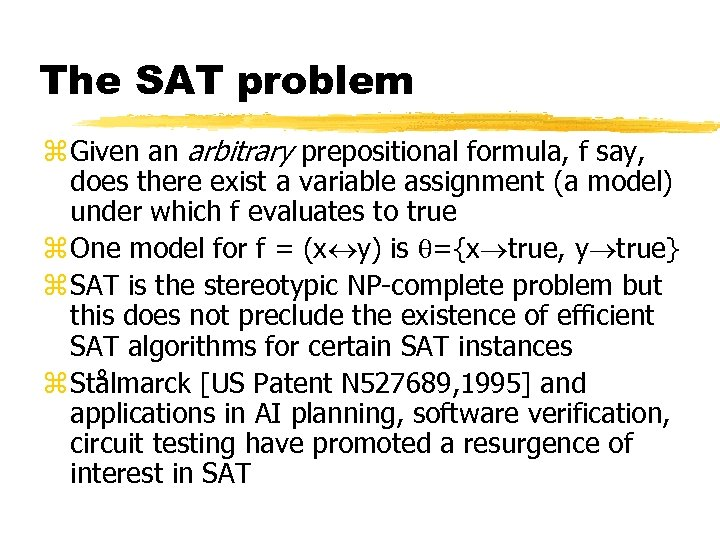The SAT problem z Given an arbitrary prepositional formula, f say, does there exist a variable assignment (a model) under which f evaluates to true z One model for f = (x y) is ={x true, y true} z SAT is the stereotypic NP-complete problem but this does not preclude the existence of efficient SAT algorithms for certain SAT instances z Stålmarck [US Patent N 527689, 1995] and applications in AI planning, software verification, circuit testing have promoted a resurgence of interest in SAT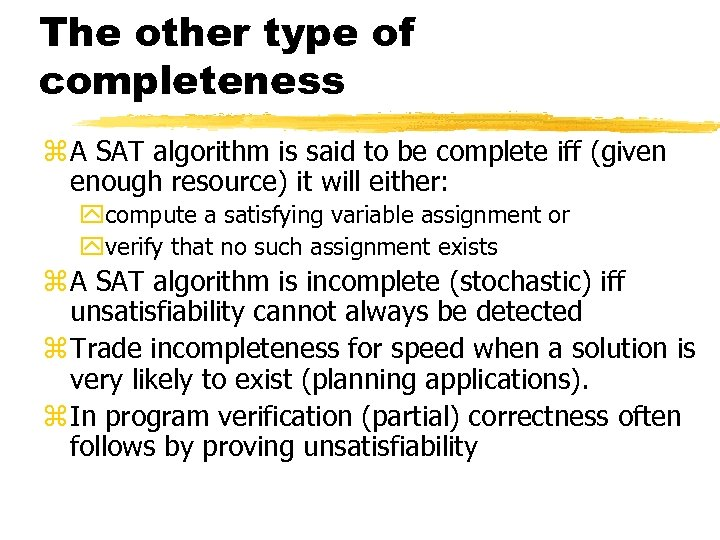The other type of completeness z A SAT algorithm is said to be complete iff (given enough resource) it will either: ycompute a satisfying variable assignment or yverify that no such assignment exists z A SAT algorithm is incomplete (stochastic) iff unsatisfiability cannot always be detected z Trade incompleteness for speed when a solution is very likely to exist (planning applications). z In program verification (partial) correctness often follows by proving unsatisfiability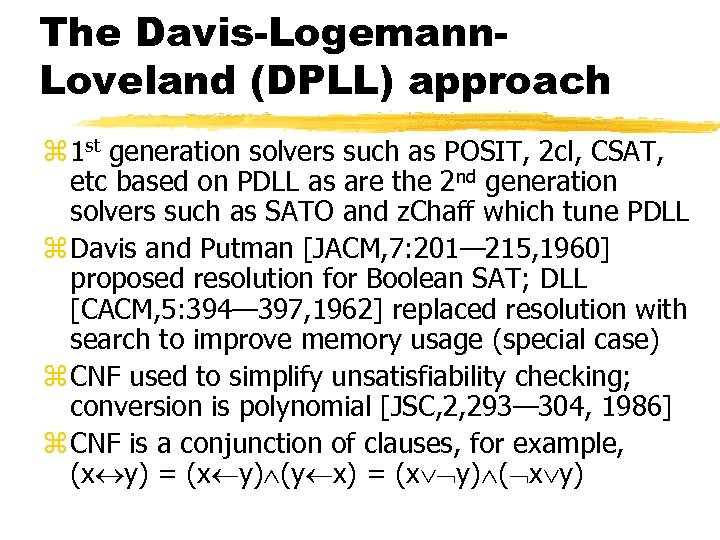The Davis-Logemann. Loveland (DPLL) approach z 1 st generation solvers such as POSIT, 2 cl, CSAT, etc based on PDLL as are the 2 nd generation solvers such as SATO and z. Chaff which tune PDLL z Davis and Putman [JACM, 7: 201— 215, 1960] proposed resolution for Boolean SAT; DLL [CACM, 5: 394— 397, 1962] replaced resolution with search to improve memory usage (special case) z CNF used to simplify unsatisfiability checking; conversion is polynomial [JSC, 2, 293— 304, 1986] z CNF is a conjunction of clauses, for example, (x y) = (x y) (y x) = (x y) ( x y)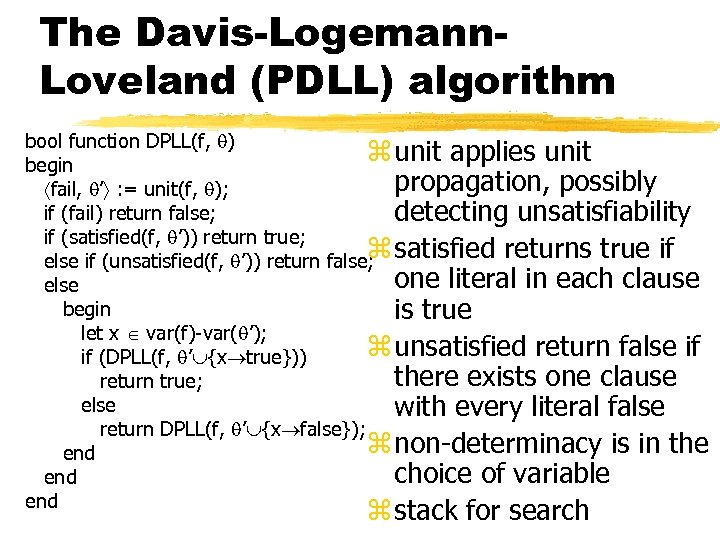The Davis-Logemann. Loveland (PDLL) algorithm bool function DPLL(f, ) z unit applies unit begin propagation, possibly fail, ’ : = unit(f, ); if (fail) return false; detecting unsatisfiability if (satisfied(f, ’)) return true; z else if (unsatisfied(f, ’)) return false; satisfied returns true if one literal in each clause else begin is true let x var(f)-var( ’); z unsatisfied return false if if (DPLL(f, ’ {x true})) there exists one clause return true; else with every literal false return DPLL(f, ’ {x false}); z non-determinacy is in the end choice of variable end z stack for search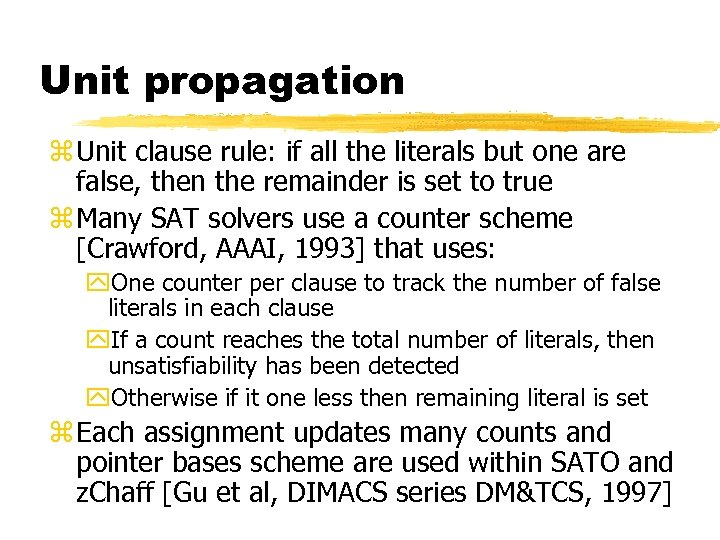Unit propagation z Unit clause rule: if all the literals but one are false, then the remainder is set to true z Many SAT solvers use a counter scheme [Crawford, AAAI, 1993] that uses: y. One counter per clause to track the number of false literals in each clause y. If a count reaches the total number of literals, then unsatisfiability has been detected y. Otherwise if it one less then remaining literal is set z Each assignment updates many counts and pointer bases scheme are used within SATO and z. Chaff [Gu et al, DIMACS series DM&TCS, 1997]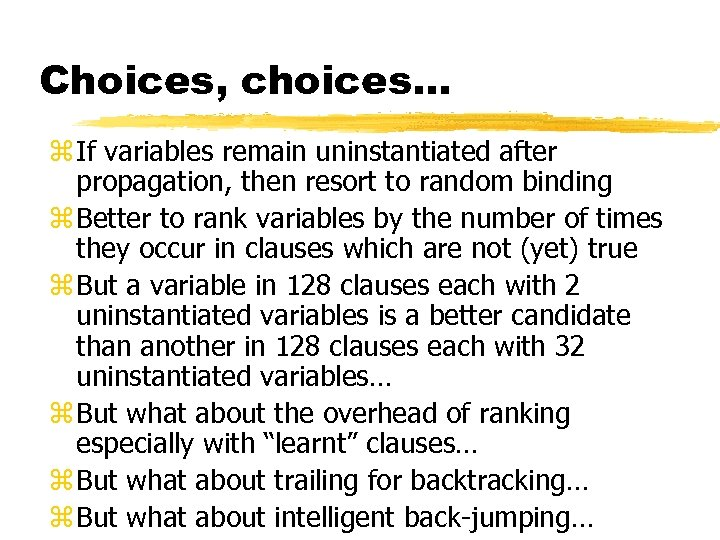Choices, choices… z If variables remain uninstantiated after propagation, then resort to random binding z Better to rank variables by the number of times they occur in clauses which are not (yet) true z But a variable in 128 clauses each with 2 uninstantiated variables is a better candidate than another in 128 clauses each with 32 uninstantiated variables… z But what about the overhead of ranking especially with “learnt” clauses… z But what about trailing for backtracking… z But what about intelligent back-jumping…